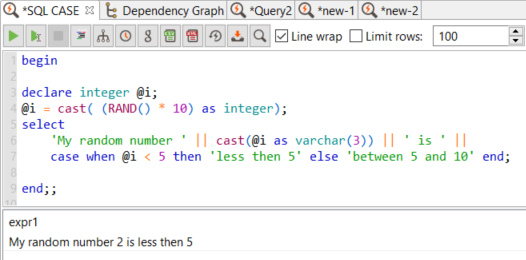Amazon Web Services AWS Tutorials and Guides Development resources, articles, tutorials, code samples and tools and downloads for ASP.Net, SQL Server, R Script, Windows, Windows Phone, AWS, SAP HANA and ABAP, like SAP UI5, Screen Personas, etc.
 Home Articles News IT Jobs Tools Sample Chapters Trainers Blogs Forums Photos Files

# SQL CASE Statement in Data Virtuality Query

For SQL developers building applications using Data Virtuality, SQL CASE statement is required frequently in View source query or in Procedure SQL codes.

When SQL programmers checks below SQL query, they can see that first of all an integer variable is declared.
Then using numeric SQL function RAND(), a random number is generated. Since Rand() random SQL function generates a double value between 0 and 1, I multiply it by 10 to generate a number between 0 and 10.
Of course a CAST conversion function is applied to convert double type data into integer type data.

Then using string concatenation expression " || ", I generate a new string value and display it as an output of the following SQL query execution.

``` begin declare integer @i; @i = cast( (RAND() * 10) as integer); select  'My random number ' || cast(@i as varchar(3)) || ' is ' ||  case when @i < 5 then 'less then 5' else 'between 5 and 10' end; end;; ```

Please pay attention to CASE statement usage.
The syntax is as follows:

``` CASE WHEN (criteria) THEN (block) ELSE (block) END ```It is possible to extend the same SQL query using CASE statement with additional WHEN clauses as follows

``` begin declare integer @i; @i = cast( (RAND() * 10) as integer); select  'My random number ' || cast(@i as varchar(3)) || ' is ' ||  case when (@i < 5) then ('less then 5') else ('between 5 and 10') end,  case @i   when (0) then ('Zero')   when (1) then ('One')   when (2) then ('Two')   when (3) then ('Three')   when (4) then ('Four')   else ('between 5 and 10')  end; end;; ```SQL developers can refer to Reference Guide for numeric functions including Rand() random number function.

For SQL expressions including CASE statement again the same Reference Guide from Data Virtuality can help more to database developers.

AWS Tutorials

AWS Tutorials

AWS